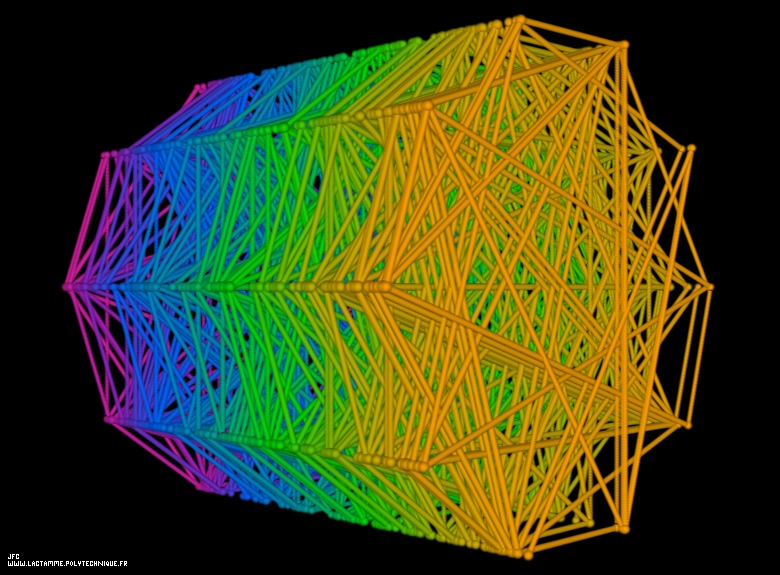The 1.000 first digits -base 10- of 'e' displayed on an helix -good point of view- [Les 1.000 premières décimales -base 10- de 'e' visualisées sur une hélice -bon point de vue-].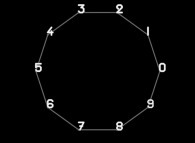To each digit D inside [0,9] is associated an angle A(D) with the following rule:
```                    A(D) = D.(2.pi/10)
```
Then each digit D(n) (n E [1,1000] for this picture) is displayed as a point (belonging to an helix) with the following tridimensional coordinates:
```                    X = cos(A(D(n)))
Y = sin(A(D(n)))
Z = n
```
At last, the picture displays all the segments {D(n),D(n+1)} (for all n).

See some related pictures (including this one):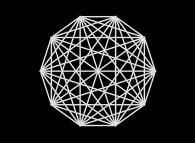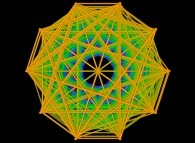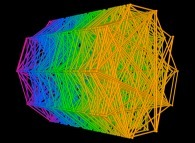The left-hand side picture seems to exhibit extraordinary symmetries, when the right-hand side one shows that there are none. Obviously, those apparent symmetries are due to the fact that 'e' should be normal number (not yet demonstrated) meaning that, for example, the frequencies of 00, 01, 02,..., 97, 98, 99 are equal (and equal to 1/100). Thus, whatever the digits p and q, one can find n such as D(n)=p and D(n+1)=q. Then all segments p ==> q must exist if a sufficient number of digits of 'pi' is computed (1000 for this picture)...

[See the 100.000 first digits -base 10- of 'e'.]

(CMAP28 WWW site: this page was created on 10/16/2018 and last updated on 08/17/2020 14:49:46 -CEST-)

[See the generator of this picture [Voir le générateur de cette image]]

[See all related pictures (including this one) [Voir toutes les images associées (incluant celle-ci)]]

[Go back to AVirtualMachineForExploringSpaceTimeAndBeyond [Retour à AVirtualMachineForExploringSpaceTimeAndBeyond]]
[The Y2K bug [Le bug de l'an 2000]]

[Site Map, Help and Search [Plan du Site, Aide et Recherche]]
[Mail [Courrier]]
[About Pictures and Animations [A Propos des Images et des Animations]]